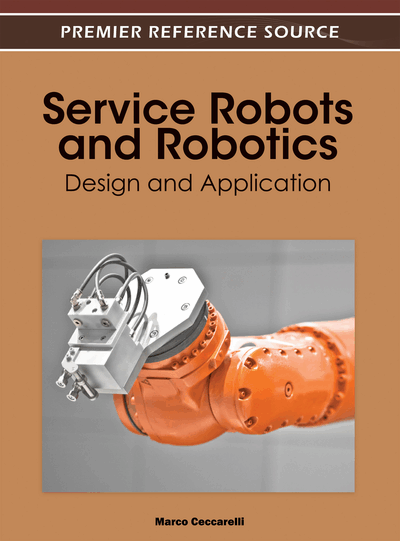# Lagrangian Dynamics of Manipulators

Sandipan Bandyopadhyay (Indian Institute of Technology, India)
DOI: 10.4018/978-1-4666-0291-5.ch005
Available
\$33.75
List Price: \$37.50
10% Discount:-\$3.75
TOTAL SAVINGS: \$3.75

## Abstract

Service robots can be thought of as having two types of motion: (a) locomotion of the entire robot, which can be either legged or wheeled, and (b) motion of the manipulator limbs, e.g., object manipulation by the “hands” etc. While the first type is very specialised, in particular in the case of legged motion, the second is fairly generic and can be discussed in detail without making a very heavy demand on the mathematical background of the reader. With that in mind, in the following, the author considers the dynamics of two types of systems, which are known as serial or open-loop, and parallel or closed-loop manipulators. The examples of these would be the hands of a humanoid robot, when considered in isolation, and when clasped together or holding an object with both hands, respectively. The examples considered here would be planar in order to keep them simple; however, the formulation presented would be general, so that the reader can, very easily, use it to model and simulate spatial manipulators.
Chapter Preview
Top

## Introduction

The purpose of this chapter is to familiarise the reader with the basics of modelling the dynamics of a robot manipulator. The framework of Lagrangian mechanics is used. The reader is expected to be conversant with the basics of robot kinematics to the extent of using Jacobian matrices in describing the velocities of various points on a robot. The fundamentals of such an approach can be found in standard textbooks, such as Craig (1986), Ghosal (2006), and Saha (2008).

The study of dynamics of a robot (or any mechanical system for that matter) can be divided into two main categories:

• Forward dynamics: This is the study of the robot’s response to a given set of inputs, i.e., forces/moments applied by the actuators at the various joints. This mode of analysis commonly forms the backbone of the synthesis of suitable control schemes for the robots, as one needs to ascertain the control responses first in simulations before applying them to the actual robot.

• Inverse dynamics: In this mode of analysis, the robot’s motion is specified, and the actuation forces1 required to cause the motion are computed. The major utility of this analysis is in sizing up appropriate actuators, and also finding out various forces in the manipulator’s links and joints that are needed for the detailed mechanical design of the manipulator, i.e., the physical dimensions of the links and joints.

As we shall see in the following, at the level of modelling the above distinction is only notional. It is only in the simulations one can choose between the modes.

Top

## Formulation Of The Equation Of Motion

The most important part of a study in dynamics is the derivation of the equation of motion. It is also known as modelling of the system, since it is the process of abstracting a mechanical system in terms of mathematical equations. Obviously, this can only be achieved (without getting into too many of the complications) if certain idealisations are incorporated in the model. The usual ones are obvious:

• 1.

The links are rigid.

• 2.

The joints are ideal.

• 3.

All the geometry and inertia parameters are known accurately.

• 4.

Frictional, as well as other disturbance forces, are either absent or their behavior is known accurately enough to be incorporated in the model.

We assume all of the above in the following. However, the reader should note that more sophisticated analysis, known as system identification, can obviate the need of the last two assumptions to some extent. Further, there is a class of robots known as flexible manipulators where the first assumption is not warranted, and the joints may not even be present!

## Complete Chapter List

Search this Book:
Reset